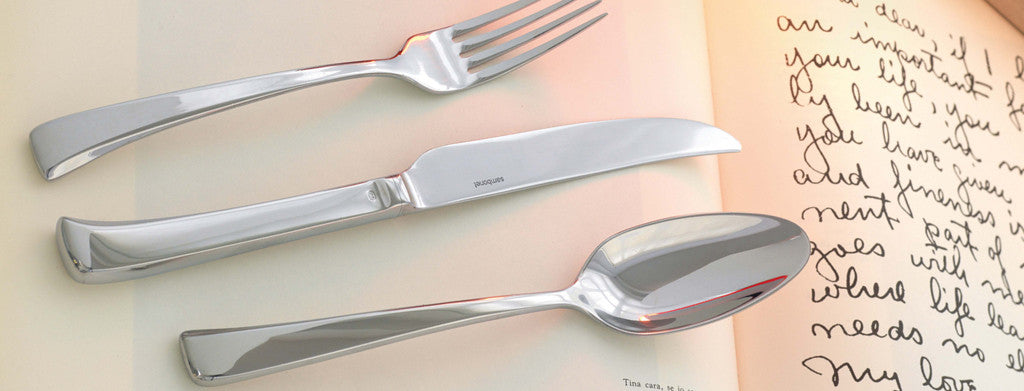Shopping Cart

### Refine

View all

#### Price# Cutlery£1.80 each (ex VAT)£1.55 each (ex VAT)£1.31 each (ex VAT)£0.29 each (ex VAT)£1.55 each (ex VAT)£0.50 each (ex VAT)£0.81 each (ex VAT)£0.32 each (ex VAT)£0.29 each (ex VAT)£0.95 each (ex VAT)£0.50 each (ex VAT)£0.48 each (ex VAT)£0.48 each (ex VAT)£0.89 each (ex VAT)£0.48 each (ex VAT)£0.69 each (ex VAT)£0.53 each (ex VAT)£0.79 each (ex VAT)£1.18 each (ex VAT)£0.50 each (ex VAT)£0.95 each (ex VAT)£0.50 each (ex VAT)£0.95 each (ex VAT)£0.48 each (ex VAT)£0.48 each (ex VAT)£0.89 each (ex VAT)£0.48 each (ex VAT)£0.32 each (ex VAT)£0.32 each (ex VAT)£0.50 each (ex VAT)£0.95 each (ex VAT)£0.42 each (ex VAT)£0.94 each (ex VAT)£1.31 each (ex VAT)£1.65 each (ex VAT)£1.31 each (ex VAT)£0.79 each (ex VAT)£1.19 each (ex VAT)£0.79 each (ex VAT)£0.69 each (ex VAT)£0.67 each (ex VAT)£1.12 each (ex VAT)£0.69 each (ex VAT)£0.41 each (ex VAT)£0.71 each (ex VAT)£0.70 each (ex VAT)£0.70 each (ex VAT)£1.14 each (ex VAT)